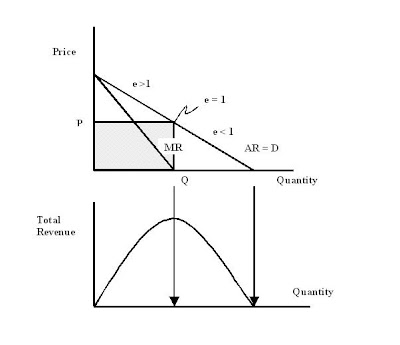## Sunday, February 13, 2011

### An Observation on Marginal Revenue and Elasticity

In the linear case: The slope of the Marginal Revenue MR curve (for a firm in imperfect competition) is twice as steep as the Average Revenue AR curve, (which is equal to the Demand curve.) This means the intersect of the MR curve with the Quantity axis is at the midpoint of the intersect of the AR curve with that axis. But this is the coordinate Q on the Quantity axis of the unit price elasticity on the Demand curve, which always lies on the midpoint of a straight line Demand curve. And this is the point where total revenue = P x Q is maximized. Thus the point of unit elasticity ( e = 1 ) is the point where total revenue is maximized. I probably read this somewhere.#### 1 comment:

1.Found this in my intorductory text in economics. I got the "probably read this somewhere" right.